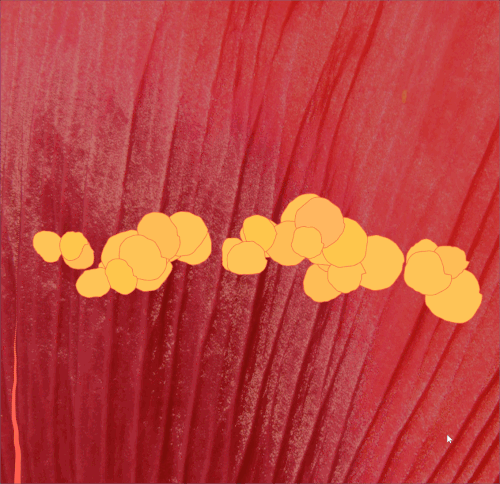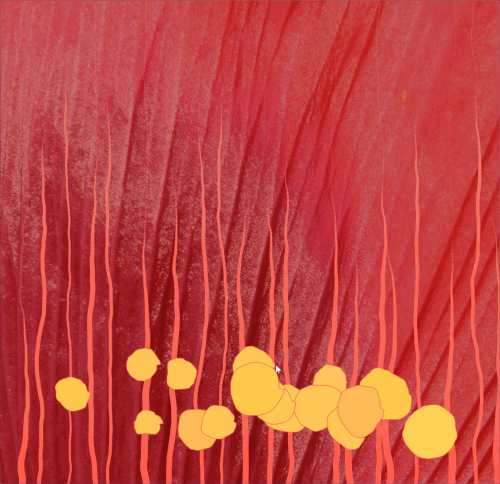# Jackalope-clock

I’ve been having some problems with anxiety recently (I think). I’d be going about my day like usual, not even thinking about anything stressful, and my chest will suddenly feel tight and my heartbeat much more noticeable and breathing suddenly a conscious effort. So this clock is to represent that, the passing of time marked by suddenly prominent body functions like breathing and heartbeat.

For the execution, I accomplished most of what I’d set out to do: bobbling blobs that represent the number of minutes into the hour and vein-like lines for the hour of the day. The blobs also travel up the screen based on the the minutes of the current time. When another minute passes, a new blob slowly grows bigger and appears (This was a bit hard to capture in the gifs due to my computer lagging a bit). A few lacking things however: I didn’t figure out a good, elegant way to add gradients to my blobs, and additionally, I had a 3D rendered model of some poppy petals that I had planned to use in the background. I wanted it as the background, and I’d also wanted to try out the different lighting options in P5.js but for the life of me, absolutely could not get the .OBJ file to load. A image of the model I made and the lighting I possibly would have wanted is attached below with sketches and gifs of the clock.Around 1:35Around 11:50Around 21:19```//special thanks to Golan Levin for the template for this clock and to Auntie P for the Poppy photo //which can be found at https://www.flickr.com/photos/auntiep/2596640834   var prevSec; var millisRolloverTime; var blobPts = []; var fullsize = false; var poppy;   function preload(){ poppy = loadImage("https://i.imgur.com/GEfCzEu.jpg"); } //-------------------------- function setup() { createCanvas(800, 800); millisRolloverTime = 0; }   //-------------------------- function draw() { background(165, 16, 7);   tint(255, 106); image(poppy, 0,0,800,800);     // Fetch the current time var H = hour(); var M = minute(); var S = second();   // Reckon the current millisecond, // particularly if the second has rolled over. // Note that this is more correct than using millis()%1000; if (prevSec != S) { millisRolloverTime = millis(); } prevSec = S; var mils = floor(millis() - millisRolloverTime);   drawLines(H, M, S, mils); drawBlobs(H, M, S, mils); }   function drawLines(H, M, S, mils) { px = width / 2.0 + 0.09 * cos(millis()); py = width / 2.0 + 0.09 * sin(millis()); var lines = H; noStroke(); for (var line = 0; line < width; line += (width / lines)) {   var radius = 0.0; for (var i = 0; i < height; i += 3) {   fill(224.0 + 0.05 * i, 129.0 - 0.05 * i, 114.0 - 0.05 * i); var maxr = 15 * noise(line); var end = height * 0.7 * noise(line); var x = line + 60 * noise(line + (millis() / 10000.0)); x += 30 * noise(line + i * 0.007); var throb = 2.0 * noise(line + mils * TWO_PI / 1000.0); var y = i; if (y > end && radius <= maxr) radius += 0.1; if (x >= 0 && x <= height) ellipse(x, y, radius + throb, radius + throb); } } }   function drawBlobs(H, M, S, mils) { var noiseScale = 0.05; var timey = map(M + S / 60.0, 0, 60, height, 0); for (var i = 0; i < M; i++) { var radius = width / 20 * noise(i * 100 * noiseScale); var x = width * noise(100000.0 * i); var y = timey + 150.0 * noise(i * 1000.0); var ybob = (10.0 * noise(i * 50.0)) * sin(TWO_PI * (S + millis() / 60.0) / 60.0); var currentBlob = []; for (var j = 0; j < 100; j++) { var rjitter = 20.0 * noise((x + y + j + millis() / 200.0) * 0.04); var throb = i / 20.0 * sin(mils * TWO_PI / 1000.0); var jitter = noise(sin(TWO_PI * j / 100.0)); if (i < M - 1 && !fullsize) { currentBlob[2 * j] = x + (radius + throb + rjitter) * cos(TWO_PI * j / 100) + jitter; currentBlob[2 * j + 1] = y + ybob + (radius + throb + rjitter) * sin(TWO_PI * j / 100) + jitter; } else { currentBlob[2 * j] = x + (min(1, millis() / 1000.0)) * ((radius + throb + rjitter) * cos(TWO_PI * j / 100) + jitter); currentBlob[2 * j + 1] = y + ybob + (min(1, millis() / 1000.0)) * ((radius + throb + rjitter) * sin(TWO_PI * j / 100) + jitter); } } blobPts[i] = currentBlob; }   for (var blob = 0; blob < M; blob++) { fill(244 + 40 * noise(blob + 1), 179 + 34 * noise(blob + 2), 66 + 40 * noise(blob + 3)); stroke(244, 104, 66); var current = blobPts[blob]; beginShape(); for (var k = 0; k < 100; k++) { vertex(current[2 * k], current[2 * k + 1]); } endShape(CLOSE); } }```